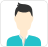true

Find the best tutors and institutes for Class 8 Tuition

Find Best Class 8 Tuition

No matching category found.

No matching Locality found.

Outside India?

Search in

# Learn Exercise 4.1 with Free Lessons & Tips

AB = 4.5 cm

BC = 5.5 cm

CD = 4 cm

AC = 7 cm

JU = 3.5 cm

UM = 4 cm

MP = 5 cm

PJ = 4.5 cm

PU = 6.5 cm

(iii) Parallelogram MORE

OR = 6 cm

RE = 4.5 cm

EO = 7.5 cm

(iv) Rhombus BEST

BE = 4.5 cm

ET = 6 cm

(i) Firstly, a rough sketch of this quadrilateral can be drawn as follows.(1) ΔABC can be constructed by using the given measurements as follows.(2) Vertex D is 6 cm away from vertex A. Therefore, while taking A as centre, draw an arc of radius 6 cm.(3) Taking C as centre, draw an arc of radius 4 cm, cutting the previous arc at point D. Join D to A and C.(ii)Firstly, a rough sketch of this quadrilateral can be drawn as follows.(1) Δ JUP can be constructed by using the given measurements as follows.(2) Vertex M is 5 cm away from vertex P and 4 cm away from vertex U. Taking P and U as centres, draw arcs of radii 5 cm and 4 cm respectively. Let the point of intersection be M.(3) Join M to P and U.(iii)We know that opposite sides of a parallelogram are equal in length and also these are parallel to each other.

Hence, ME = OR, MO = ER

A rough sketch of this parallelogram can be drawn as follows.(1) Δ EOR can be constructed by using the given measurements as follows.(2) Vertex M is 4.5 cm away from vertex O and 6 cm away from vertex E. Therefore, while taking O and E as centres, draw arcs of 4.5 cm radius and 6 cm radius respectively. These will intersect each other at point M.(3) Join M to O and E.MORE is the required parallelogram.

(iv)We know that all sides of a rhombus are of the same measure.

Hence, BE = ES = ST = TB

A rough sketch of this rhombus can be drawn as follows.(1) Δ BET can be constructed by using the given measurements as follows.(2) Vertex S is 4.5 cm away from vertex E and also from vertex T. Therefore, while taking E and T as centres, draw arcs of 4.5 cm radius, which will be intersecting each other at point S.(3) Join S to E and T.BEST is the required rhombus.Swapna Shree

How can we Improve it?

Please enter your question below and we will send it to our tutor communities to answer it *

Name *

Enter a valid name.

Email *

Enter a valid email.

Email or Mobile Number: *

UrbanPro.com helps you to connect with the best Class 8 Tuition in India. Post Your Requirement today and get connected.

X

### Looking for Class 8 Tuition Classes?

Find best tutors for Class 8 Tuition Classes by posting a requirement.

• Post a learning requirement
• Get customized responses
• Compare and select the best### Looking for Class 8 Tuition Classes?

Find best Class 8 Tuition Classes in your locality on UrbanPro